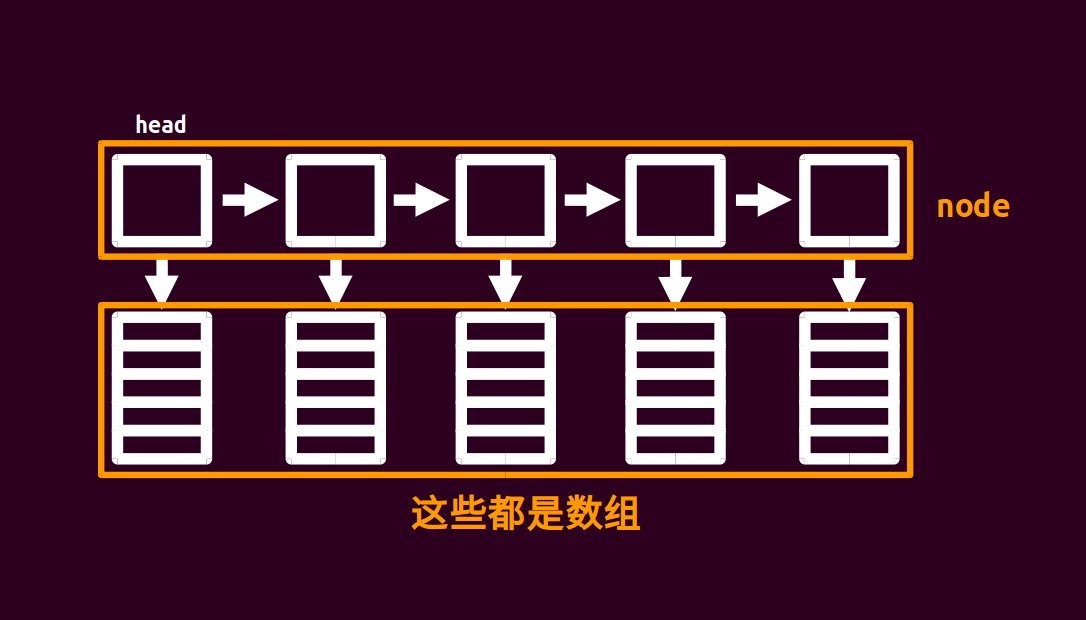# 块状链表1 2 3 4 5 6 7 8 9 struct node { node* nxt; int size; char d[(sqn << 1) + 5]; node() { size = 0, nxt = NULL, memset(d, 0, sizeof(d)); } void pb(char c) { d[size++] = c; } }; 

 1 list > orz_list; 

## STL 中的 rope

### 导入

STL 中的 rope 也起到块状链表的作用，它采用可持久化平衡树实现，可完成随机访问和插入、删除元素的操作。

 1 2 #include using namespace __gnu_cxx; 

OI 中，关于能否使用双下划线开头的库函数曾经一直不确定，2021 年 CCF 发布的 关于 NOI 系列活动中编程语言使用限制的补充说明 中提到「允许使用以下划线开头的库函数或宏，但具有明确禁止操作的库函数和宏除外」。故 rope 目前可以在 OI 中正常使用。

### 基本操作

rope <int > a初始化 rope（与 vector 等容器很相似）
a.push_back(x)a 的末尾添加元素 x
a.insert(pos, x)apos 个位置添加元素 x
a.erase(pos, x)apos 个位置删除 x 个元素
a.at(x)a[x]访问 a 的第 x 个元素
a.length()a.size()获取 a 的大小

## 例题

POJ2887 Big String

  1 2 3 4 5 6 7 8 9 10 11 12 13 14 15 16 17 18 19 20 21 22 23 24 25 26 27 28 29 30 31 32 33 34 35 36 37 38 39 40 41 42 43 44 45 46 47 48 49 50 51 52 53 54 55 56 57 58 59 60 61 62 63 64 65 66 67 68 69 70 71 72 73 74 75 76 77 #include #include #include using namespace std; static const int sqn = 1e3; struct node { // 定义块状链表 node* nxt; int size; char d[(sqn << 1) + 5]; node() { size = 0, nxt = NULL; } void pb(char c) { d[size++] = c; } }* head = NULL; char inits[(int)1e6 + 5]; int llen, q; void readch(char& ch) { // 读入字符 do ch = getchar(); while (!isalpha(ch)); } void check(node* p) { // 判断，记得要分裂 if (p->size >= (sqn << 1)) { node* q = new node; for (int i = sqn; i < p->size; i++) q->pb(p->d[i]); p->size = sqn, q->nxt = p->nxt, p->nxt = q; } } void insert(char c, int pos) { // 元素插入，借助链表来理解 node* p = head; int tot, cnt; if (pos > llen++) { while (p->nxt != NULL) p = p->nxt; p->pb(c), check(p); return; } for (tot = head->size; p != NULL && tot < pos; p = p->nxt, tot += p->size) ; tot -= p->size, cnt = pos - tot - 1; for (int i = p->size - 1; i >= cnt; i--) p->d[i + 1] = p->d[i]; p->d[cnt] = c, p->size++; check(p); } char query(int pos) { // 查询 node* p; int tot; for (p = head, tot = head->size; p != NULL && tot < pos; p = p->nxt, tot += p->size) ; tot -= p->size; return p->d[pos - tot - 1]; } int main() { scanf("%s %d", inits, &q), llen = strlen(inits); node* p = new node; head = p; for (int i = 0; i < llen; i++) { if (i % sqn == 0 && i) p->nxt = new node, p = p->nxt; p->pb(inits[i]); } char a; int k; while (q--) { readch(a); if (a == 'Q') scanf("%d", &k), printf("%c\n", query(k)); else readch(a), scanf("%d", &k), insert(a, k); } return 0; }